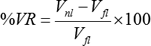##Voltage Regulation Improve in Transmission Lines

If we consider how the high voltage in the transmission lines should regulate, then the answer is with a transformer.

But if we consider how the voltage is increased in an already operating transmission line this can be done by couplers in the transformer in or decreasing the number of turns in the transformer, thereby raising or lowering the voltage.

Under heavy load conditions, more current drawn from your TL and this increases the voltage drop V=IZ and to increase the voltage at the sending end there are some methods that can be used:

Method-1 changes Z, where method-2 changes current I. And these are what voltage drop depends on Vdrop=IZ. Decreasing voltage drop means raising the voltage at receiving end.

1. You can add series capacitors to your TL with each phase at some distances of your line. The capacitive reactance Xc will remove part of the inductive reactance Xl and hence the total impedance Z will decrease. And when Z decrease that means your voltage will rise because the voltage drop now is small.
2. Using shunt capacitors, using shunt capacitors at receiving end or at selected points across your TL will compensate part of the inductive reactance, and hence will decrease the current that flows in the TL and this will decrease the voltage drop and hence increases the voltage. These shunt capacitors, in fact, act like power factor correctors, they increase pf and then decrease the current: I=P3√∗V∗pf as you see when pf increases the current will decrease from this equation.

In most cases, the shunt capacitors are used at the receiving end of the TL in the substations.

### How to Improve Voltage Regulation in Power Distribution Line

Mainly controlling the voltage level in distribution system voltage regulation is improved. The possible way to improve voltage regulation in electrical power distribution system network is as below:

1. Use of generator voltage regulators;

2. Application of voltage-regulating equipment in the distribution substations;

3. Application of capacitors in the distribution substation;

4. Balancing of the loads on the primary feeders;

5. Increasing of feeder conductor size;

6. Changing of feeder sections from single-phase to multiphase;

7. Transferring of loads to new feeders;

8. Installing of new substations and primary feeders;

9. Increase of primary voltage level;

10. Application of voltage regulators on the primary feeders;

11. Application of shunt capacitors on the primary feeders;

12. Application of series capacitors on the primary feeders;

### How is voltage regulation calculated?

Voltage Regulation for 11KV, 22KV, 33KV Overhead Line
• % Voltage Regulation = (1.06 x P x L x PF) / (LDF x RC x DF)
• RC = (KV x KV x 10) / ( RCosΦ + XSinΦ)
• Required size of Capacitor (Kvar) = KVA1 (Sin ø1 – [Cos ø1 / Cos ø2] x Sin ø2)
• L = [1 – (KVARC / 2 KVARL) x (2n – 1)]
• % Voltage Rise = (KVAR(Cap) x Lx X) / 10 x Vx2

#### Nominal Valu of Voltage Regulation:

• Above 33kV (-) 12.5% to (+) 10%.
• Up to 33kV (-) 9.0% to (+) 6.0%.
• Low voltage (-) 6.0% to (+) 6.0%

### What is Voltage Regulation in Power System

By definition voltage regulation is “the ratio of voltage drop from no load to the full load to the no-load voltage”.

There are two ways to express the voltage regulation:

One is voltage regulation up and another is voltage regulation down.

Consider the general formula for voltage regulation up as

Here Vnl is the no-load voltage and Vfl is the full load voltage.

The voltage regulation up is normally used in the case of transformers.

Consider the general formula for voltage regulation down asThe voltage regulation is normally used in the case of alternators and power system calculations and its efficiency scaling.

1.2.